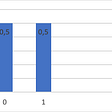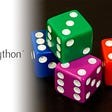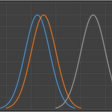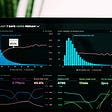# Statistics: Empirical distribution function

This is part of the course “Probability Theory and Statistics for Programmers”.

Let’s assume that we studying some random variable X with unknown distribution law and we need to find one. In order to find this distribution law, we need to make some number of independent experiments over this random variable. Results of these experiments are simple statistical series. By using this data we can make empirical distribution function. This cumulative function is a step function that jumps up by 1/n at each of the n data points. Its value at any specified value of the measured variable is the fraction of observations of the measured variable that are less than or equal to the specified value. In order to find the value of empirical distribution function for specific value x, we should calculate the number of experiments in which random variable X had a value less than x and divide on a total number of experiments.

Let’s take a look at an example. We roll the dice 100 times. How will look empirical distribution function?

By increasing the number of experiments empirical distribution function comes closer to the real distribution function.

In the previous example, the function looks close to the real distribution function. But if we increase the number of experiments we can obtain a much better result.

If X is a continuous random variable, by increasing the number of experiments we increase the number of function steps, so the step of function decrease and empirical function approaches a smooth curve — real distribution function of random variable X. I have shown this in the article about distribution functions.

Next part ->

Reach the next level of focus and productivity with increaser.org.

--

--

--

Indie hacker behind increaser.org. More at radzion.com

Love podcasts or audiobooks? Learn on the go with our new app.

## Don’t Stop Me Now## Mathematical Economics: The Good and The Bad## Discrete random variable distributions | Probability theory | Part 4## Probability theory: Basic concepts## What is Money?## Loss and Percentage Profit Calculation Formulas## Formal Systems, Self Reference, and EpimenidesIndie hacker behind increaser.org. More at radzion.com

## ANOVA with Python## Python Pandas — Reading Data and Calculating Correlation## Count The Number of Moon Rocks Using Python